# 4 Dominance Constraints

Trees are widely used for representing hierarchically organized information, such as syntax trees, first-order terms, or formulae representing meanings. A tree can be regarded as a particular type of directed graph. A directed graph is a pair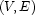where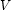is a set of vertices and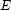a multiset of directed edges between them, i.e. a subset of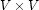. A forest is an acyclic graph where all vertices have in-degree at most 1. A tree is a forest where there is precisely one vertex, called the root, with in-degree 0; all others have in-degree 1.

First-order terms, or finite constructor trees, are characterized by a further restriction: each node is labeled by some constructorof a signature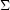, and its out-edges are labeled by integers from 1 to n, where n is the arity of the constructor. This can be formalized as follows: we assume a signatureof function symbols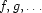, each equipped with an arity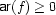. A finite constructor tree is then a triple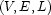wheredefines a finite tree, and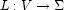and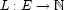are labelings, and such that for any vertex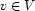there is exactly one outgoing edge with labelfor each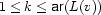and no other outgoing edges.

Trees are often used to represent syntactic structure. For example, here is a possible analysis of "beans, John likes everyday".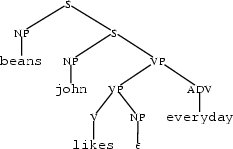or logical formulae representing meanings. For example, here are the simplified representations of the two meanings of the ambiguous sentence "every yogi has a guru":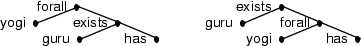Denys Duchier
Version 1.2.0 (20010221)